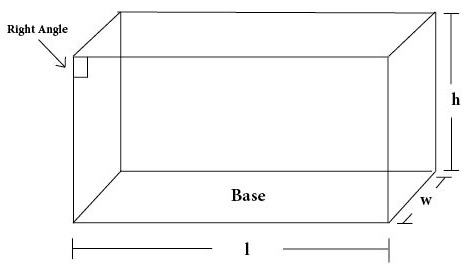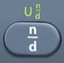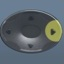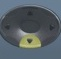## Right Rectangular Prism

##### Right Rectangular Prism = Three-Dimensional Shape in which Opposite Faces are Equal (with all right angles)Basics

l = length
w = width
h = height
p = perimeter of base
B = area of base
Base = floor of prism
SA = Surface Area = area covering all surfaces of prism (measured in square units)
V = Volume = space occupied by prism (measured in cubic units)

A cube is a special version of a right rectangular prism in which all faces are equal.

Formulas (provided by Mathematics Formula Sheet)

SA = ph + 2B
V = Bh

Formulas (NOT provided by Mathematics Formula Sheet)

p = 2l + 2w
B = lw
h = V ÷ B

Question
A cinder block has a length of 100 centimeters, width 60 centimeters, and height 80 centimeters.  What is its surface area?

37600 square centimeters

p = 2l + 2w
p = 2 × 100 + 2 × 60 = 320

B = lw
B = 100 × 60 = 6000

SA = ph + 2B
SA = 320 × 80 + 2 × 6000 = 37600

Calculator Click What You See Commentblinker clears screen
2 × 100 + 2 × 60 2*100+2*60 p = 2l + 2w320 p
100 × 60 100*60 B = lw6000 B
320 × 80 + 2 × 6000 320*80+2*6000 SA = ph + 2B37600 Answer
###### Right Rectangular Prism

Question
With reference to the question above, what is the cinder block’s surface area in square meters?

3.76 square meters

See Measurement.
Square Meters = Square Centimeters ÷ 10000
Square Meters = 37600 ÷ 10000

Calculator Click What You See Commentblinker clears screen
2 × 100 + 2 × 60 2*100+2*60 p = 2l + 2w320 p
100 × 60 100*60 B = lw6000 B
320 × 80 + 2 × 6000 320*80+2*6000 SA = ph + 2B37600
37600 ÷ 10000 37600÷10000 Square Meters =
Square Centimeters ÷ 100003.76 Answer
###### Right Rectangular Prism

Question
A cinder block has a length of 100 centimeters, width 60 centimeters, and height 80 centimeters.  What is its volume?

480000 cubic centimeters

B = lw
B = 100 × 60 = 6000

V = Bh
V = 6000 × 80 = 480000

Calculator Click What You See Commentblinker clears screen
100 × 60 100*60 B = lw6000 B
6000 × 80 6000*80 V = Bh480000 Answer
###### Right Rectangular Prism

Question
With reference to the question above, what is the cinder block’s volume in cubic meters?

0.48 cubic meters

See Measurement.
Cubic Meters = Cubic Centimeters ÷ 1000000
480000 ÷ 1000000 = 0.48

Calculator Click What You See Commentblinker clears screen
100 × 60 100*60 B = lw6000 B
6000 × 80 6000*80 V = Bh480000
480000 ÷ 1000000 480000÷1000000 Cubic Meters =
Cubic Centimeters ÷ 10000000.48 Answer
###### Right Rectangular Prism

Question
A toy block has a length of$\bf\displaystyle10\frac{1}{2}$ centimeters, width$\bf\displaystyle6\frac{1}{2}$ centimeters, and height$\bf\displaystyle8\frac{1}{2}$ centimeters.  To the nearest hundredth of a cubic centimeter, what is its volume?

580.13 cubic centimeters

B = lw

B =$\bf\displaystyle 10\frac{1}{2}$*$\bf\displaystyle 6\frac{1}{2}$$\bf\displaystyle\frac{273}{4}$

V = Bh

V =$\bf\displaystyle\frac{273}{4}$*$\bf\displaystyle 8\frac{1}{2}$$\bf\displaystyle\frac{4641}{8}$ = 580.125 after toggle = 580.13 after rounding

Calculator Click What You See Commentblinker clears screen1012×612$\bf\displaystyle 10\frac{1}{2}$*$\bf\displaystyle 6\frac{1}{2}$ B = lw$\bf\displaystyle\frac{273}{4}$ B2734×812$\bf\displaystyle\frac{273}{4}$*$\bf\displaystyle 8\frac{1}{2}$ V = Bh$\bf\displaystyle\frac{4641}{8}$580.125 Answer
(before rounding)
###### Right Rectangular Prism

Question
A cinder block has a length of 120 centimeters, width 50 centimeters, and volume 780000 cubic centimeters.  What is its height?

130 centimeters

B = lw
B = 120 × 50 = 6000

h = V ÷ B
h = 780000 ÷ 6000 = 130

Calculator Click What You See Commentblinker clears screen
120 × 50 120*50 B = lw6000 B
780000 ÷ 6000 h = V ÷ B130 Answer
###### Right Rectangular Prism

Practice – Questions
1.  A cinder block has a length of 50 centimeters, width 30 centimeters, and height 40 centimeters.  What is its surface area?

2.  With reference to Question 1, what is the cinder block’s surface area in square meters?

3.  A cinder block has a length of 50 centimeters, width 30 centimeters, and height 40 centimeters.  What is its volume?

4.  A toy block has a length of$\bf\displaystyle5\frac{1}{2}$ centimeters, width$\bf\displaystyle3\frac{1}{2}$ centimeters, and height$\bf\displaystyle4\frac{1}{2}$ centimeters.  To the nearest hundredth of a cubic centimeter, what is its volume?

5.  A cinder block has a length of 60 centimeters, width 25 centimeters, and volume 67500 cubic centimeters.  What is its height?